Published on

# Assignment Operators in python

Authors
•Name
Aswin Barath
@AswinBarath2

Assignment operators are used to assigning values to variables.

That is to store values in variables we use `=` assignment operator.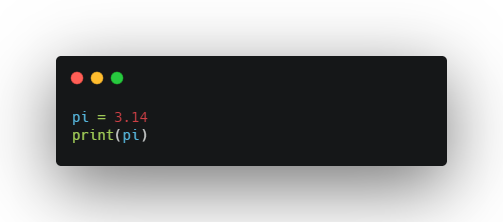Output:

``````3.14
``````

OK, now comes the real fun. Have ever been tired to use `x = x + 5`, where we type the variable x twice? There's actually a shortcut for this called Augmented assignment operators.

Augmented assignment operators can be used as a replacement as follows:

``````x += 3	   --->	   x = x + 3
x -= 3	   --->	   x = x - 3
x *= 3	   --->	   x = x * 3
x /= 3	   --->	   x = x / 3
x %= 3	   --->	   x = x % 3
x //= 3	   --->	   x = x // 3
x **= 3	   --->	   x = x ** 3
x &= 3	   --->	   x = x & 3
x |= 3	   --->	   x = x | 3
x ^= 3	   --->	   x = x ^ 3
x >>= 3	   --->	   x = x >> 3
x <<= 3	   --->	   x = x << 3
``````

## Here's the Code and Output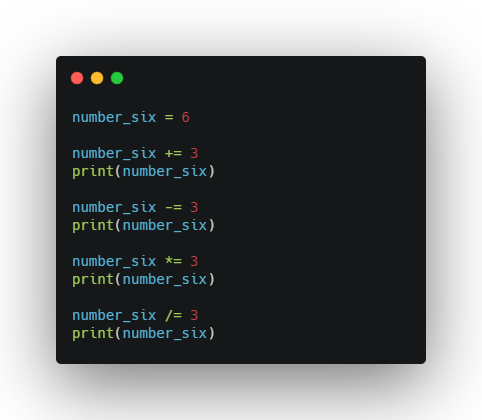``````9
6
18
6.0
``````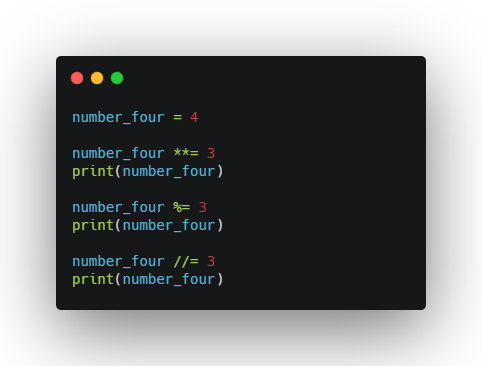``````64
1
0
``````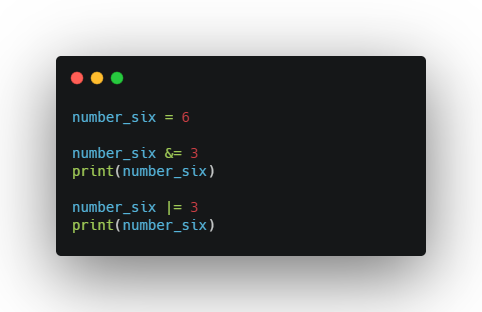``````2
3
``````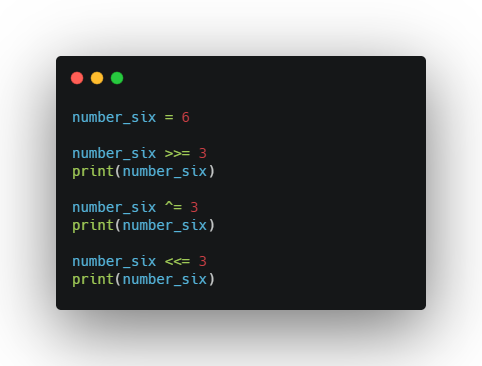``````0
3
24
``````

Quick Note: The code snippets reuses the same variable to assign with different arithmetic operations / bitwise operations / shift operations.

So, while coding makes sure you practice to use print statements after each operation.